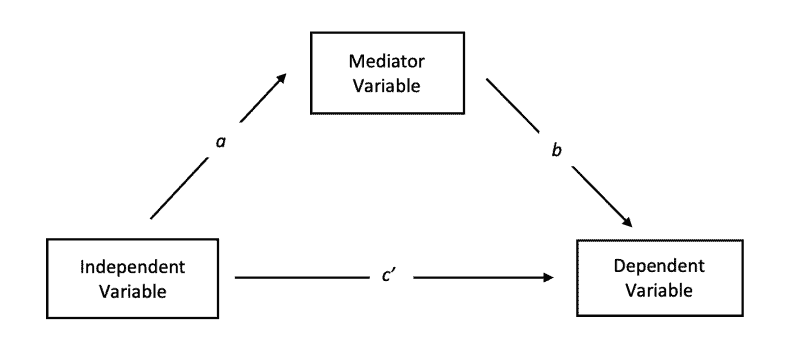Statistics

# Variable and Its MeasurementBy variable we mean the characteristics which can be measured; Image source: Wikimedia commonsWritten by

Variables

By variable in research we mean the characteristics which can be measured numerically and the value of which varies from individuals to individuals, item to item. It can also call a Quantitative Variable. Example: For human beings height, weight, number of siblings, sex, and marital status are all example of variables.

The characteristic which can be measured but not expressed numerically and which indicates a difference in the quality of the phenomenon is known as an attribute. It can also define as Qualitative Variable. Example: Efficiency (Efficient/inefficient), gender, eye color are attributes.

Discrete Variable

A quantitative variable whose possible values are a finite (or countable infinity) set of numbers is called a discrete variable. Example: The number of children in each family of Dhaka city is a discrete variable.

Continuous Variable

A quantitative variable whose possible values are some interval of numbers is called a continuous variable. Example: Age of human beings, speed of automobiles etc.

Data

Information attained by observing the values of a variable is data. Data is a plural word that comprehends the idea of the collection of pieces of information. The singular word is a datum.

Data Set

Each individual piece of data is called observation and the collection of all observations of a particular investigation is called a data set.

Measurement scales

It is very important because it relates to the types of statistics you can use to analyze your data. There are four types of measurement levels used in statistical analysis and researchers can use a combination of all or use specific levels to gauge any characteristics such as 1. Nominal. 2. Ordinal, 3. Interval, 4. Ratio

Nominal

The lowest measurement level from a statistical point of view is a nominal scale. This scale, as the name implies, is simply some placing of data into categories, without any order or structure. In research activities, a YES/NO scale is a very common example of a nominal scale. It has no order and there is no distance between YES and NO. The nominal scales belong in a non-parametric group. In order to analyze nominal data, we can estimate mode and cross-tabulation – with chi-square statistics.

Ordinal

An ordinal scale is next up the list in terms of the scale of measurement. The simplest ordinal scale is ranking. When a researcher asks you to rank the top 5 Universities of the country, he is asking you to create an ordinal scale of preference. The difference between ordinal and nominal scale is an ordinal scale used to arrange data into order rather than nominal scale. Ordinal data would use non-parametric statistics. This analysis would include:

1. Median and mode, 2. Rank order correlation, 3. Non-parametric analysis of variance

Interval

By Interval scale we mean a standard survey rating scale. When you are asked to rate your satisfaction with training on a 7 point scale, from Dissatisfied to Satisfied, you are using an interval scale. It is an interval scale because it is assumed to have central points between each of the scale elements. This means that it is possible to interpret differences in the distance along the scale. In contrast, this to an ordinal scale where we can only talk about differences in order, not differences in the degree of order.

Interval scales are also defined by metrics such as logarithms. In these cases, the distances are not equal but they are strictly definable based on the metric used. In order to analysis Interval scale data would use parametric statistical techniques:

1. Mean and standard deviation, 2. Correlation – r, 3. Regression, 4. Analysis of variance, 5. Factor analysis, 6. Advance multivariate analysis

It should be mention; you can use non-parametric techniques with interval and ratio data. But non-parametric techniques are less powerful rather the parametric ones.

Ratio

A ratio scale is the top level of measurement scale and is not often obtainable in social research. A ratio scale starts from a true zero point. In other words, we can say that when zero means the absence of the characteristics then the appropriate scale to use is the ratio scale. The simplest example of a ratio scale is the measurement of weight. The analysis is the same as for Interval data.

Comparative discussion on different scales of measurement

The following table can be constructed from the definition of the different scales of measurements.

### About the author#### Rifat Afroze

Rifat Afroze is a Staff Researcher of the Research and Evaluation Division of BRAC, Dhaka, Bangladesh.